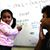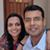D

#### What is the practical use of calculating jerk?

71 viewed last edited 1 year agoAnonymous
0

A jerk (third derivative of displacement with respect to time) is easy to calculate, but I cannot identify a practical use for it.

Could you provide a scenario where this calculation is used?S. Prem Kumar
4

'Jerk' as you have referred to is the third derivative of the displacement with respect to time or the first derivative of the acceleration. In most problems that you may have encountered acceleration (such as g, due to gravity) is taken to be constant. This is of course only an idealisation. In the real world acceleration changes all the time.

Take for example motion of a car on a busy road, as it moves, stops, and then accelerates and decelerates. For such non-uniform motion you would expect that all derivatives of displacement are generally non-zero (being sloppy with vector signs):

\frac{dx}{dt} = velocity

\ \frac{d^2x}{dt^2} = acceleration

\ \frac{d^3x}{dt^3} = jerk

\frac{\ d^4x}{dt^4} = snap

\frac{\ d^5x}{dt^5}. = crackle

^{\frac{d^6x}{dt^6}}= pop

etc.

You can give names to higher derivatives as you wish. You would naturally ask: Why would these be useful ? In any real motion, as in the case of a car on a busy road or motion of a roller coaster or complex movement of machine parts, these derivatives of displacement and indeed even the higher ones will be non-zero. The more jerky the motion, the more the number of non-zero derivatives.

The jerk which is the rate of change of acceleration is important because engineers want to make this as small as possible in order to make your car/train or roller-coaster ride as comfortable as possible, and more importantly to ensure passenger safety. The human body cannot withstand jerks or changes in acceleration beyond a certain point without sustaining damage (whiplash injuries). This is also true for machine parts. Railway lines which are curved and then straighten out are designed in ways to minimise the change in the acceleration as the train comes out of the curved stretch into the straight section.  Here is a link to a very nice little paper which explains all the different facets of changes in acceleration and their practical implications:

http://iopscience.iop.org/article/10.1088/0143-0807/37/6/065008Mahesh Godavarti
00
Remember the good old steam locomotive? Those had much higher jerk while starting, so it was hard to sit still when they started to move. Modern electric locomotives have a much lower jerk.Vivekanand Vellanki
2

jerk is defined as the rate of change of acceleration; as you point out the 3rd derivative with respect to time.

You would not have seen any equations using jerk - and, that is the reason you are asking this question.

One very important thing to note: The equations

• v\ =u+at and
• s=ut+\frac{1}{2}at^2and
• v^2-u^2=2as

apply for uniform acceleration - that is the magnitude and direction of acceleration do not change.

When jerk is not 0, the above equations do not hold. One has to come up with new equations of motion. One way of doing that is to differentiate the above equations and replace \frac{da}{dt} by jerk.

For problems involving objects falling towards the earth, the assumption is that g (acceleration due to gravity) is constant. However, this is not true, the gravitational force depends on the distance and as the distance reduces the force increases - hence acceleration increases.

For simplicity, the effect of such changes in the acceleration are ignored. However, there may be cases where we cannot ignore such changes in the acceleration.